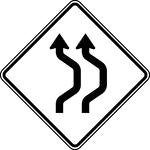### Double Reverse Curve, Black and White

The Double Reverse Curve sign may be used when the tangent distance between two reverse curves is less…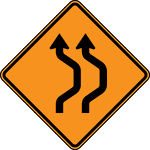### Double Reverse Curve, Color

The Double Reverse Curve sign may be used when the tangent distance between two reverse curves is less…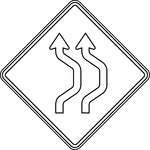### Double Reverse Curve, Outline

The Double Reverse Curve sign may be used when the tangent distance between two reverse curves is less…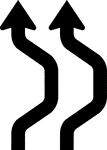### Double Reverse Curve, Silhouette

The Double Reverse Curve sign may be used when the tangent distance between two reverse curves is less…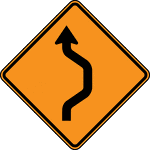### Single Reverse Curve, Color

The Double Reverse Curve sign may be used when the tangent distance between two reverse curves is less…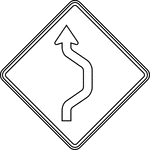### Single Reverse Curve, Outline

The Double Reverse Curve sign may be used when the tangent distance between two reverse curves is less…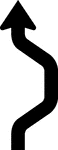### Single Reverse Curve, Silhouette

The Double Reverse Curve sign may be used when the tangent distance between two reverse curves is less…### Single Reverse Curve, Black and White

The Double Reverse Curve sign may be used when the tangent distance between two reverse curves is less…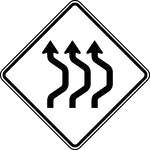### Triple Reverse Curve, Black and White

The Double Reverse Curve sign may be used when the tangent distance between two reverse curves is less…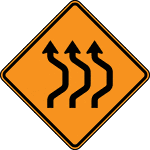### Triple Reverse Curve, Color

The Double Reverse Curve sign may be used when the tangent distance between two reverse curves is less…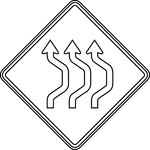### Triple Reverse Curve, Outline

The Double Reverse Curve sign may be used when the tangent distance between two reverse curves is less…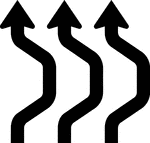### Triple Reverse Curve, Silhouette

The Double Reverse Curve sign may be used when the tangent distance between two reverse curves is less…